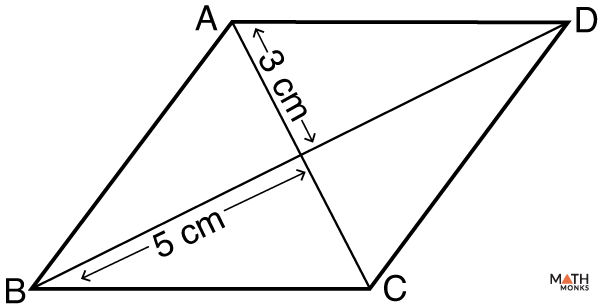# Area of Rhombus

The area of a rhombus is the total space enclosed by the rhombus on a two-dimensional plane. The unit for the area is square units, such as sq. cm, sq. m, sq. ft, etc.

## Formulas

There are 3 ways to find the area of a rhombus based on the parameter(s) given. The formulas are given below.

### Using Diagonals

This is the most common method used to find the area of a rhombus. The formula to calculate the area of a rhombus when the diagonals are known is given below:

#### Derivation

Let us take a rhombus ABCD where O is the point of intersection of two diagonals d1 and d2.

∆AOB is a right triangle as the diagonals of a rhombus are perpendicular bisectors.

The area of the rhombus will be 4 × area of ∆AOB. So the equation to derive the formula is written as:

= 4 × (½) × AO × OB

= 4 × (½) × (½) d1 × (½) d2

= 4 × (1/8) d1 × d2

= ½ × d1 × d2

Let us solve an example to understand the concept better.

Calculate the area of a rhombus having diagonals equal to 9 inches and 8 inches.

Solution:

As we know,
A = ½ × d1 × d2, here d1 = 9 inches, d2 = 8 inches
= ½ × 9 × 8
= 36 sq. inchesWhat is the area of rhombus ABCD.

Solution:

As we know,
A = ½ × d1 × d2, here d1 = 2 ×3 = 6 cm, d2 = 2 × 5 = 10 cm (∵ half of diagonals are 3 cm & 5 cm)
= 6 × 10
= 60 sq. cm

### Using Base and Height

The formula to calculate the area of a rhombus when the base and height are known is given below:

Let us solve an example to understand the concept better.

Finding the area of a rhombus when BASE and HEIGHT are known

What is the area of the rhombus with a base of 15 cm and a height of 11 cm?

Solution:

As we know,
A = b × h, here b = 15 cm, h = 11 cm
= 15 × 11
= 165 sq. cm

### Using Side and Vertex Angle

The formula to calculate the area of a rhombus when side and any vertex angle are known is given below:

Let us solve an example to understand the concept better.

Finding the area of a rhombus when SIDE and VERTEX ANGLE are known

Find the area of a rhombus whose side is 18 cm and one of its angles is 30°.

Solution:

As we know,
A = s2 × sin(θ), here s = 18 cm, θ = 30°
= 182 × sin(30)
= 324 × ½
= 162 sq. cm